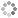# PROWAREtechWorking with the `Task` class in .NET is practically identical to working with the `Thread` class (see related article) with one important difference — the .NET asynchronous functions support the `CancellationToken` for cancelling a thread which means it is mildly easier to work with tasks.

1. Tasks can return a result whereas thread functions/methods are are always `void` return type
3. Tasks automatically implement cancellation tokens (threads can implement cancellation tokens, too)

.NET Tasks use threads but wraps them in classes to make them a higher level concept. Tasks seem similar to Intel Threading Building Blocks.

#### Example of a Task Returning a Value

``````using System;

namespace ConsoleApp1
{
class Program
{
{
string s = await Task.Run(async () => // async not necessary in this example
{
return (args.Length > 0 ? args : "no arguments"); // VALUE RETURNED ON THIS LINE
});
Console.WriteLine(s);
}
}
}
``````

#### Example of Waiting for Tasks to Finish

Tasks are added to a `List` and then a special method, `Task.WhenAll`, holds execution until all tasks are finished. This is easily manipulated to work with classes containing a `Task` member through either a Select statement or simply looping through all the Tasks checking `Task.IsCompleted`.

``````using System.Collections.Generic;

namespace ConsoleApp1
{
class Program
{
{
for (int i = 0; i < 20; i++)
{
{
for (int x = 0; x < 1000000000; x++) ;
}));
}
}
}
}
``````

### Find π on Each Logical Processor Using Tasks

This version of finding pi does so with floating point variables. See this article for an example of using .NET threading to solve pi with integers. `FindPi_2` is the popular Bailey–Borwein–Plouffe formula.

``````using System;
using System.Collections.Generic;
using System.Linq;

namespace ConsoleApp1
{
{
public int id { get; }
public double pi { get; set; }
public ulong iterations { get; set; }
public CancellationTokenSource CancelToken { get; }
{
var spaces = Environment.ProcessorCount.ToString().Length;
this.id = id;
CancelToken = new CancellationTokenSource();
iterations = (ulong)new Random().Next() * 10;
Console.WriteLine("Starting Job: {0, -" + spaces + "} Iterations: {1}", id, iterations);
task = Task.Run(async () => // async not necessary in this example
{
if((id & 1) == 0)
pi = FindPi_2(iterations, CancelToken);
else
pi = FindPi_1(iterations, CancelToken);
Console.WriteLine("Job: {0, -" + spaces + "} ended with pi={1}", id, pi.ToString("0.00000000000000")); // apply some fancy formatting
}, CancelToken.Token);
}

static double FindPi_1(ulong count, CancellationTokenSource cancel)
{
//π = 3.14159265358979323846264338327950288419...
//π = (4/1) - (4/3) + (4/5) - (4/7) + (4/9) - (4/11) + (4/13) - (4/15) + ...
//π = 4 * (1 - 1/3 + 1/5 - 1/7 + 1/9 - 1/11 + 1/13 - 1/15 + ...)

double x = 1.0;
for (ulong i = 2; !cancel.IsCancellationRequested & i < count; i++)
x += ((i & 1) == 0 ? -1.0 : 1.0) / ((i << 1) - 1);
return 4.0 * x;
}

static ulong Power(ulong x, ulong y)
{
ulong m = 1;
for (ulong i = 0; i < y; i++)
m *= x;
return m;
}
static double FindPi_2(ulong count, CancellationTokenSource cancel) // this is the fast, efficient and accurate Bailey–Borwein–Plouffe formula
{
//π = 3.14159265358979323846264338327950288419...

double x = 0.0;
for (ulong k = 0, p = Power(16, k); (p > 0) & (k < count); k++, p = Power(16, k))
x += (4.0 / (8.0 * k + 1) - 2.0 / (8.0 * k + 4) - 1.0 / (8.0 * k + 5) - 1.0 / (8.0 * k + 6)) / p;
return x;
}
}
class Program
{
static void Main(string[] args)
{
Console.WriteLine("pi={0}", 3.1415926535897932384626433832795028841971693993751M.ToString("0.00000000000000"));
Console.WriteLine("Logical Processors: {0}", Environment.ProcessorCount);
Console.WriteLine("ENTER A JOB NUMBER TO TERMINATE IT AT ANYTIME");

var spaces = Environment.ProcessorCount.ToString().Length;

int[] jobsIds = new int[Environment.ProcessorCount];
for (int i = 0; i < Environment.ProcessorCount; i++)
jobsIds[i] = i;

foreach (var jobId in jobsIds)

{
Environment.Exit(0);
});

while (jobTasks.Where(j => j.task.IsCompleted == false).Count() > 0) // look for a request to cancel a job from the user
{
var id = Console.ReadLine(); // this is blockingThis site uses cookies. Cookies are simple text files stored on the user's computer. They are used for adding features and security to this site. Read the privacy policy.